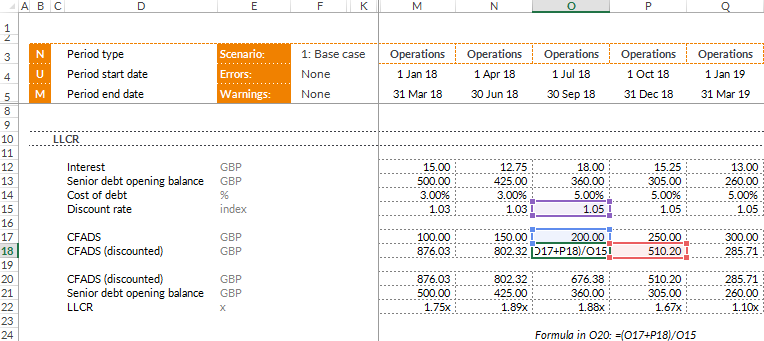Where the DSCR captures this at a single point in time, the LLCR is a longer term measurement – it looks at the period spanning the whole loan life.

It is also a ratio that frequently appears as a covenant required by the lenders in project financing. A ratio of 1.0x means that the LLCR is at break-even level. The project company’s cash flow is just enough to pay off its debt at the end of the debt tenor. Important to note is that this is irrespective of the DSCR falling below 1.0x in certain periods.

Equally, a ratio of 2.0x means that at any point in time, the discounted value of future cash flows (CFADS) is twice that of the debt balance outstanding.

### HOW IS THE LLCR CALCULATED?

LLCR is calculated by taking the net present value of CFADS over the course of the (remaining) loan life and dividing this by the debt balance outstanding:

LLCR = NPV (CFADS over the loan life) / Debt balance outstanding

Let us break this down:

• CFADS over the loan life: This is exactly what it sounds like – you simply sum the discounted CFADS over the (remaining) loan life.
• Debt balance outstanding: It is important to note that it is always the opening debt balance that is used. The purpose is to see if the project company can make the scheduled repayments, and consequently, repayments cannot be included.

### THE PURPOSE OF THE LLCR

Firstly, the LLCR is less sensitive than the DSCR since it is essentially a discounted average and not exposed to the periodical changes that the DSCR highlights.

Secondly, the LLCR is the most meaningful at the start of the loan life. This is since the lenders normally want to know whether the total amount of forecast cash flow covers the total amount of debt, not taking into account the fluctuations that the DSCR can be exposed to.

### WHAT DISCOUNT RATE TO USE?

What discount rate to use is often over-complicated and so I won’t elaborate further than to say that the discount rate used is more often than not calculated as: Total Interest and Financing fees / Opening debt balance.

There are some complexities that arise with calculating the LLCR though – mainly if your interest rate is changing over time. Note that if you are calculating the LLCR in each period over the course of the loan, the discount rate will change period to period. Whereas I would normally always advise to use the XNPV function over the NPV function, with varying interest rates you will need to calculate the discounted values in each period.*Example of discounting a cash flow with a varying discount rate

Note that this method will replicate the NPV function in that it also discounts the first value in the range. The implication here being that the value in M18 will be as of 1 Jan 18.

### CLOSING REMARKS

Always check the term sheets for the exact definition of LLCR as it can vary. A good example is that the DSRA is sometimes taken into account and sometimes excluded.

As previously mentioned, some of the most common Project Finance ratios are the Debt Service Coverage Ratio (DSCR), the Loan Life Coverage Ratio (LLCR) and the Project Life Coverage Ratio (PLCR). What these ratios have in common is the purpose of analysing the project company’s ability to repay its debt. How they relate to and complement each other will be covered in more detail at a later stage.

### NEXT STEPS

This is a summary explaining another of the most common project finance ratios, and also the fourth part in the series of Project Finance Basics. Be sure to keep up to date and follow the coming parts, which eventually will be summarised in a Whitepaper – covering the most commonly used terms in Project Finance modelling.